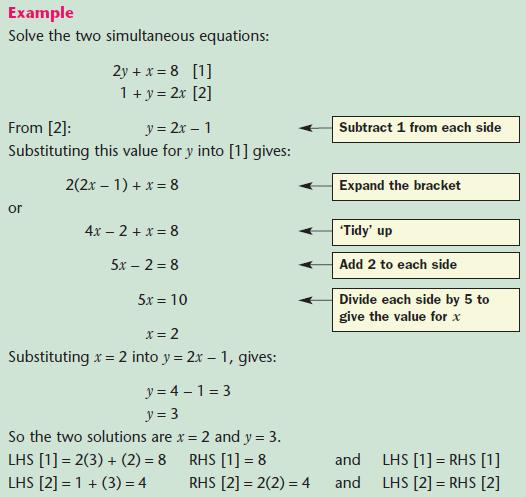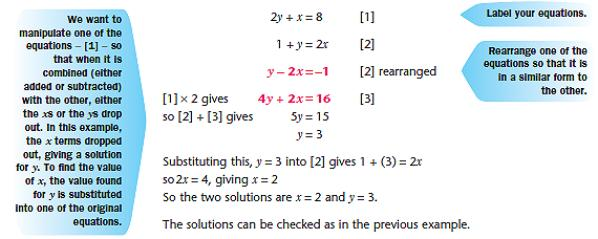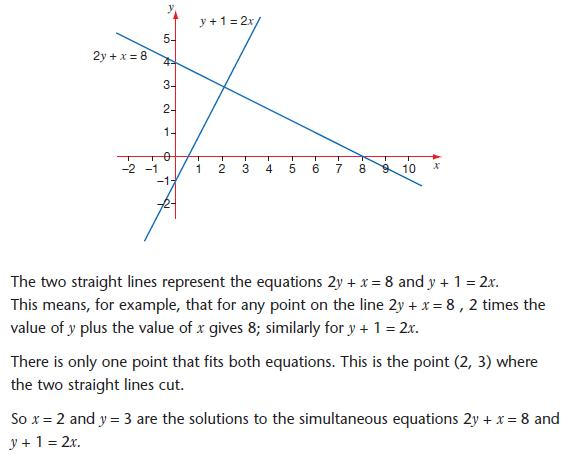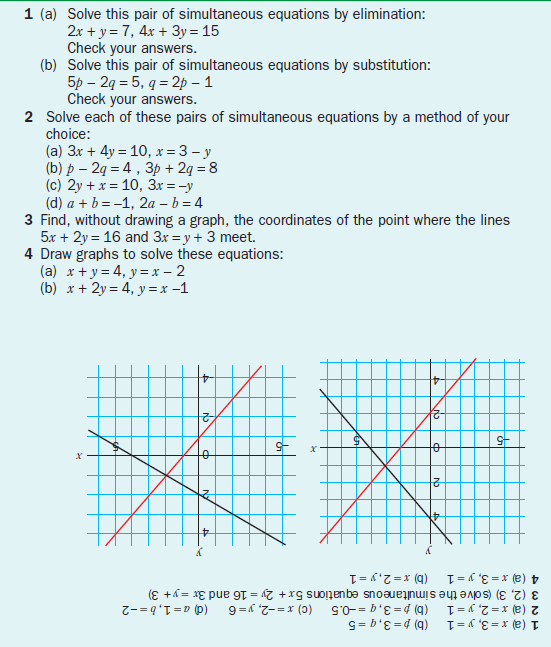## Title

Simultaneous Linear Equations
Quick revise

After studying this section, you will be able to:

• solve simultaneous linear equations by substitution
• solve simultaneous linear equations by elimination
• solve simultaneous linear equations using straight line graphs

If an equation has two unknowns, such as 2y + x = 20, it cannot have unique solutions. Two unknowns require two equations which are solved at the sametime (simultaneously) − but even then two equations involving two unknowns do not always give unique solutions.

The video below works through examples of simultaneous equations. The step-by-step example shows how to group like terms and then add or subtract to remove one of the unknowns, to leave one unknown to be solved.This method is called solution by substitution.
It involves what it says − substitution − using one of the equations to get an expression of the form ‘y = …’ or ‘x = …’ and substituting this into the other equation. This gives an equation with just one unknown, which can be solved in the usual way. This value is then substituted in one or other of the original equations, giving an equation with one unknown.

NOTE:With this method you need to take particular care with your algebra. Should your solutions be ‘strange’ fractions such as 9/13 the chances are you’ve  made a slip − check your algebra.NOTE:

• It is a good idea to label each equation. It helps you explain what you are doing − and may gain you method marks.
• This value of x can be substituted into equation  or , or into the expression for y: y = 2x − 1.
• Choose the one that is easiest!
• As a check, substitute the values back into each of the two starting equations.

The second method is called solution by elimination.

NOTE:The method is not quite as hard as it first seems, but it helps if you know why it works.

It works because of two properties of equations:

• Multiplying (or dividing) the expression on each side by the same number does not alter the equation.
• Adding two equations produces another valid equation:
e.g. 2x = x + 10 (x = 10) and x − 3 = 7 (x also = 10).
Adding the equations gives 2x + x − 3 = x + 10 + 7 (x also = 10).

The object is to manipulate the two equations so that, when combined, either the x term or the y term is eliminated (hence the name) − the resulting equation with just one unknown can then be solved:Solving simultaneous linear equations using straight line graphsNOTE:
Because the graphs of 2y + x = 8 and y + 1 = 2x are straight lines, they are called linear equations.

Drawing two straight line graphs to solve simultaneous
equations also works the other way round − solving the two simultaneous equations algebraically gives the coordinates of the point of intersection of the two straight line graphs of the two simultaneous equations.

PROGRESS CHECKRate: